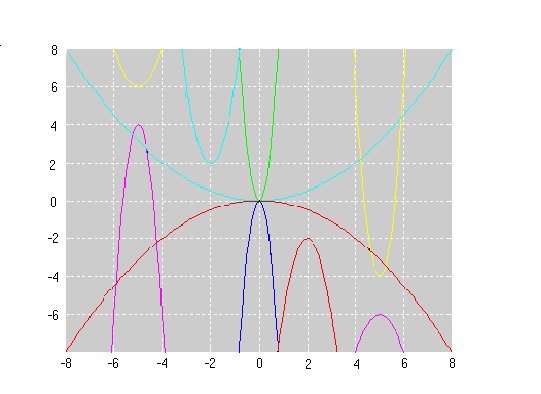#### You may also like### Cubic Spin

Prove that the graph of f(x) = x^3 - 6x^2 +9x +1 has rotational symmetry. Do graphs of all cubics have rotational symmetry?### Sine Problem

In this 'mesh' of sine graphs, one of the graphs is the graph of the sine function. Find the equations of the other graphs to reproduce the pattern.### Parabolic Patterns

The illustration shows the graphs of fifteen functions. Two of them have equations y=x^2 and y=-(x-4)^2. Find the equations of all the other graphs.

# Parabolas Again

##### Age 14 to 18Challenge LevelHere is another family of graphs sent in by Andrei from Rumania together with his solution to this problem. Can you find the equations of the graphs in Andrei's illustration?

These challenges, together with the two challenges Parabolic Patterns and More Parabolic Patterns , develop the ideas of transformations of graphs and the corresponding equations.

The illustrations give families of graphs, all of parabolas, formed by reflections in the $x$ and $y$ axes and in the line $y=x$, together with translations and stretches parallel to the axes.

You are not expected to plot and join points although it is helpful to consider where the graphs cut the axes. Rather you should think of the features of the graphs and the effects of the transformations.